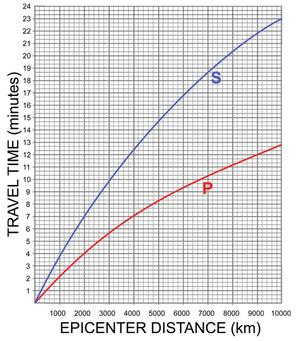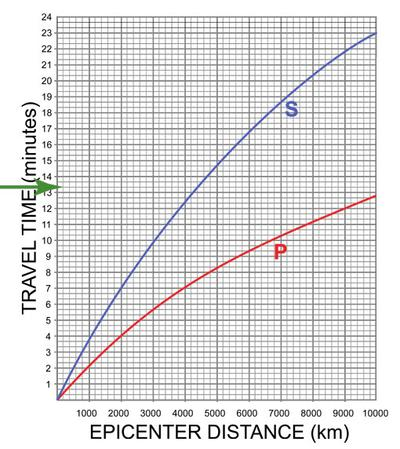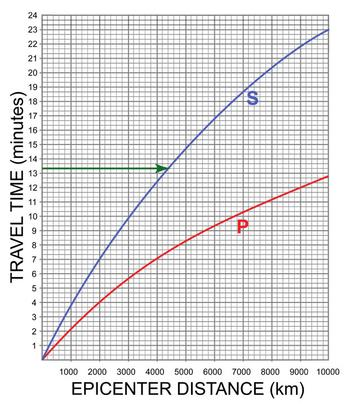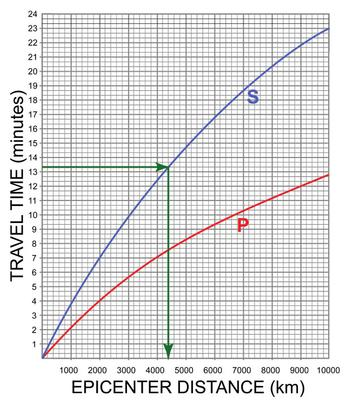# How do I read a point from a curve or line?Reading data from a graph

Sometimes, in introductory geoscience courses, you are asked to read data from a graph (the graph may have a line or a curve on it). You may have constructed the line or curve yourself (for more information see constructing a best fit line) or it may be a graph that was constructed for you. Either way, the procedure is the same for finding a data point. A quick review of the basics of graphing might help - particularly the tutorial on plotting points since the basic idea comes from this concept.

## When should I read a point from a graph?

If you are given a value for one of the parameters (or variables) on the graph and asked to determine the value of the other, the question is asking you to read a point from the plot. Remember that variables will be labeled on the axes of the graph (e.g., Epicenter distance and travel time on the graph at the right).In the plot to the right - showing P- and S- wave travel times through the Earth, the x-axis is labeled 'EPICENTER DISTANCE' and the y-axis is labeled 'TRAVEL TIME'. Thus, if you are given a travel time for the P-wave, you should be able to determine the distance to the epicenter. For example, if I tell you that it took 7 minutes for the P-wave (the red curve) to arrive, by the time you finish reading through this page, you should be able to tell me that the epicenter of the earthquake was 4000 km away.

## How do I read a point from a graph with a curve or line?

Let's use the plot to the right as an example for reading data from a graph. This plot shows travel times as related to distance for P-waves (red curve) and S-waves (blue curve) from the epicenter of an earthquake. P- and S-waves are two types of waves that are generated by earthquakes; P-waves travel faster than S-waves. If you would like a PDF to print out so that you can look at the full sized image, you can download one here (Acrobat (PDF) 149kB Jun19 09).

### Some simple steps for reading data from a graph

There are a few simple steps for reading a point from a line (or curve). We will use the following example to illustrate our steps:

The S-wave takes 13 minutes and 20 seconds to arrive at our measuring station. How far away is the epicenter?
1. Begin by making sure you know the scale and units on each of the axes.
Begin by looking at the axes. On the plot to the right, the x-axis (horizontal axis) is labeled EPICENTER DISTANCE (km) and each bold line is 1000 km. The y-axis (vertical axis) is labeled TRAVEL TIME (minutes) and each bold line is 1 minute.
2. Look at the scale of each of the axes.
The plot to the right shows the P- and S-wave plot zoomed in to show the increments.

On the x-axis of this plot, the increments between each (vertical) bold line are 1000 km (this is a major increment on the plot). Between each bold line, there are five increments. To figure out how many km between each minor increment, divide the major increment by the the number of smaller increments. So, 1000 divided by 5 gives us 200 km for each smaller increment.

The y-axis is a bit more complicated because the scale is measured in time. In this case, the vertical axis is labeled minutes and each bold (horizontal) line represents 1 minute. Remember that each minute is divided up into 60 seconds (not 10 or 100). Thus, 60 divided by the three increments between each minute gives us 20 seconds each.
3. Next, determine which axis shows the data you have been given in the question. Find the given value on that axis.
In the question, you are told that the S-wave takes 13 minutes and 20 seconds to arrive. Which of the axes provides information in minutes (and seconds)? The y-axis! Keeping in mind the scale, find 13 min 20 sec on the y-axis.

You can click on the plot to make it bigger. Note that the arrow is pointing to the line just above the one marked 13. This is because each minor mark represents 20 seconds, so the mark above 13 represents 13 minutes and 20 seconds.4. Draw a line (horizontally if you know y or vertically if you know x) from the point you marked on the axis until it hits the curve or line you are interested in.
In this case, you know the y value and you are asked to read from the S-wave curve (the blue line). Follow a horizontal line from 13 min 20 sec until it intersects the blue line.

Note the green arrow that extends from the y axis until it intersects with the blue curve (click to enlarge).5. Now, you can find the answer by drawing a straight line from where your line from step 4 intersects the curve to the other axis.

Because you know the y-value, you want to read off the x-axis (which happens to be in units of distance - kilometers). Draw a line straight down until it intersects the x-axis and read the number that is there - 4400 km (click to enlarge). Remember that each increment on the x-axis is 200 km. The line intersects the x-axis 2 units past 4000 km, so the epicenter must be 4400 km away.This is a very simple example of how we read data from plots and graphs. The concepts presented here are the same as those used to read and understand information on plots with fields of data (e.g., metamorphic rocks, sediment carrying capacity, etc.) Please visit the sample problems page for more examples and practice!

## Where is graph reading used in the geosciences?

Graph reading can be found throughout the geosciences in a variety of contexts. There are very few topics in introductory geoscience that do not include graph reading of some sort. Some of the more common uses of generating data from a graph, or reading points from a graph include: